# The Quantum Way

``````---+-----+-----
1 | 1   |{73}
---+-----+-----
2 |{74} | 94
---+-----+-----
3 | 95  | 113
---+-----+-----
4 |{114}| 121
---+-----+-----
5 | 122 | 135
---+-----+-----
6 | 136 | 156
---+-----+-----
7 |{157}|{165}
---+-----+-----
``````

What is critical to understand, is that the invisible hand of 2, 3 and 5, and their factorial 30, create the structure within which the balance of the prime numbers, i.e., all those greater than 5, are arrayed algorithmically–as we shall demonstrate.

In number theory, the partition function p(n) represents the number of possible partitions of a non-negative integer n. For instance, p(4) = 5 because the integer 4 has the five partitions 1 + 1 + 1 + 1, 1 + 1 + 2, 1 + 3, 2 + 2, and 4. (Wikipedia).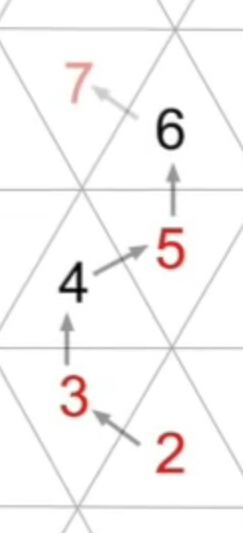The integer thirty (30) is the 19th composite number, Organizing Principle of the Prime Number Sequence, provides both vertical and horizontal structure to the prime number sequence in Prime Spiral Sieve.

The number 30 possesses remarkable attributes, including–and perhaps most profoundly–its role (along with its prime factors 2, 3 and 5) as a primary organizing principle in the distribution of prime numbers (Primesdemystified).

``````           15
↓
29
↓
---+-----+-----
6 | 30  | 31   ------
---+-----+-----       |
7 | 32  | 44         |
---+-----+-----       | 4th (id:12)
8 | 45  | 46         |
---+-----+-----       |
9 | 47  | 49   --329-¤-multiplication zone
---+-----+-----       | 5th (id:13)
10 | 50  | 50   ------
---+-----+-----
11 | 51  | 53   ------
---+-----+-----       |
12 | 54  | 59         |
---+-----+-----       | 6th (id:14 - id:15)
13 | 60  | 82         |
---+-----+-----       |
14 | 83  |{102} --289-¤-exponentiation zone
---+-----+-----       | 7th (id:16 - id:19)
15 |{103}| 110  ------
---+-----+-----
``````

Prime hexagon is a mathematical structure developed by mathematician T. Gallion that is formed when integers are sequentially added to a field of tessellating equilateral triangles, where the path of the integers is changed whenever a prime is encountered.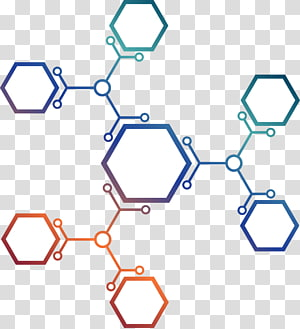The tessellating field of equilateral triangles fills with numbers, with spin orientation flipping with each prime number encountered, creating 3 minor hexagons, assigned names blue, red and green.Many of you with familiarity with Docker for Windows know how you currently have to switch between running either Windows or Linux Containers. Prime hexagon teaches us how to combat this limitation and run both simultaneously on the same host.

## Separated segments

Functional description of DNA, useful e.g. for identifying new coding sequences, comprises assigning characteristic values to individual bases derived from vibrational properties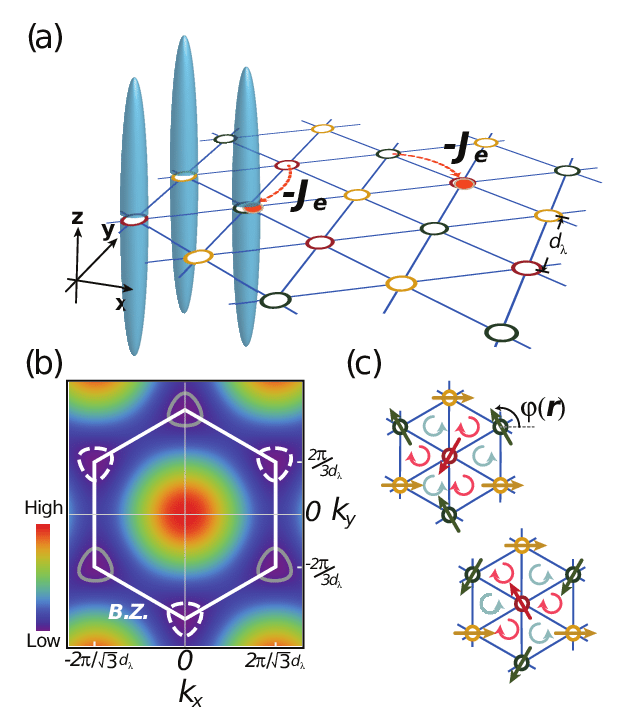The lagging strand is the strand of new DNA whose direction of synthesis is opposite to the direction of the growing replication fork. The lagging strand is synthesized in short, separated segments.

320 + 289 + 168 = 618 + 168 = 786

Cell types are interesting, but they simply reflect a modulo six (6) view of numbers. More interesting are the six internal hexagons within the Prime Hexagon. Like the Prime Hexagon, they are newly discovered.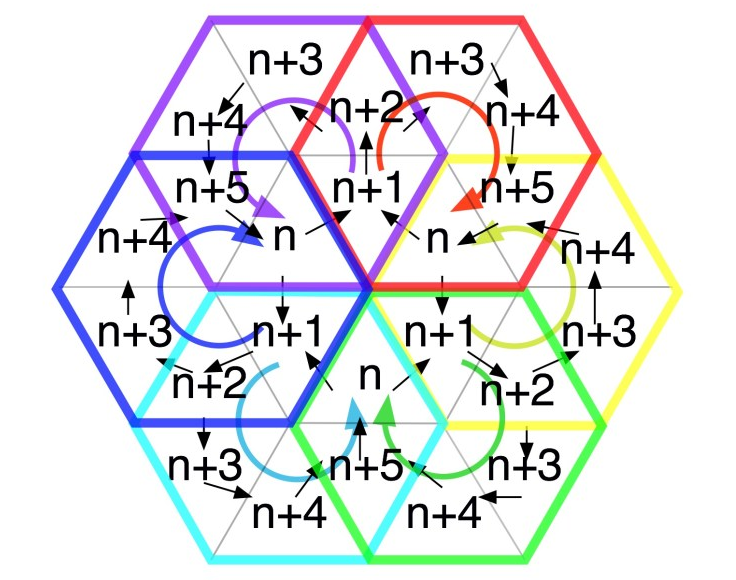Surprisingly, the 24-cell hexagon confines all natural numbers. The reason: no prime numbers occupy a cell with a right or left wall on the t-hexagon's outer boundary, other than 2 and 3.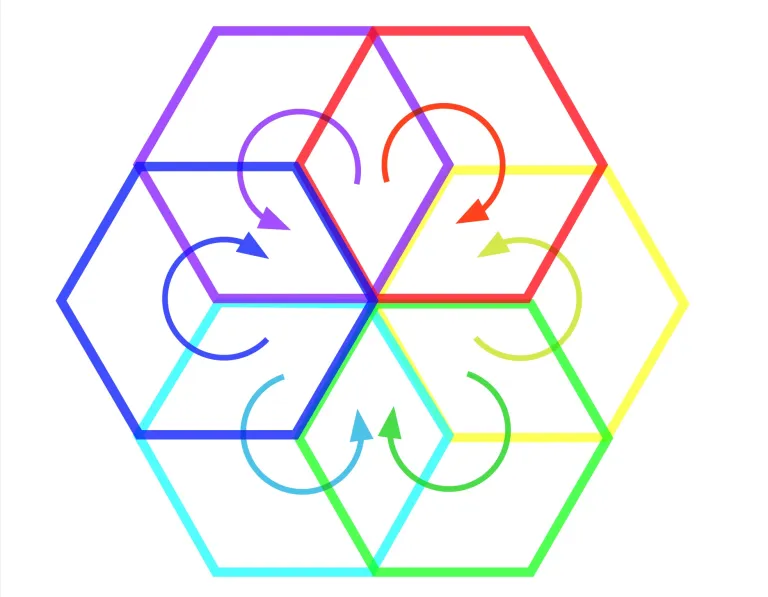### Three dimensional sphere

In 1904 the French mathematician Henri Poincaré asked if the three dimensional sphere is characterized as the unique simply connected three manifold. This question, the Poincaré conjecture, was a special case of Thurston's geometrization conjecture.

Perelman's proof tells us that every three manifold is built from a set of standard pieces, each with one of eight well-understood geometries (ClayMath Institute).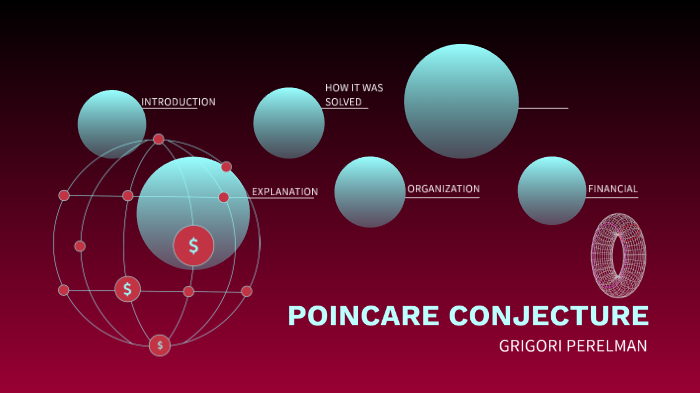The initial primes that forced the number line into this complex coil. Without a prime number in the outer set of triangles, the number line does not change to an outward course and remains forever contained in the 24 cells shown above.

The minor hexagons form solely from the order, and type, of primes along the number line. Perhaps they have no significance. however, as they do form I suspect they must connect to deeper things in mathematics (Prime Hexagon).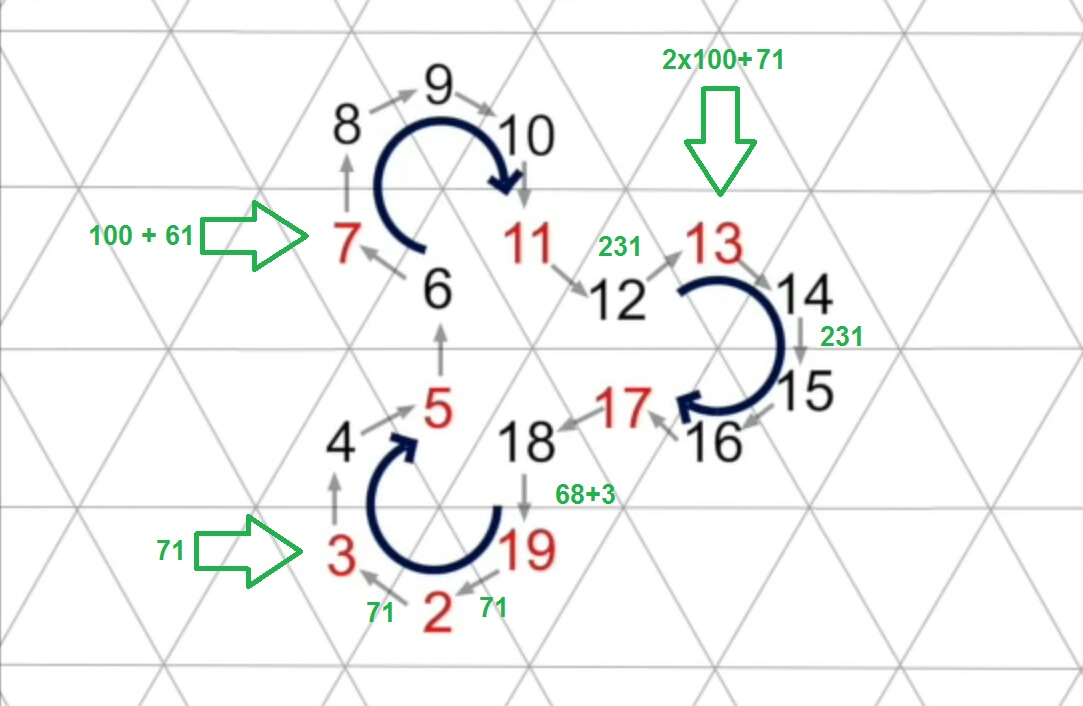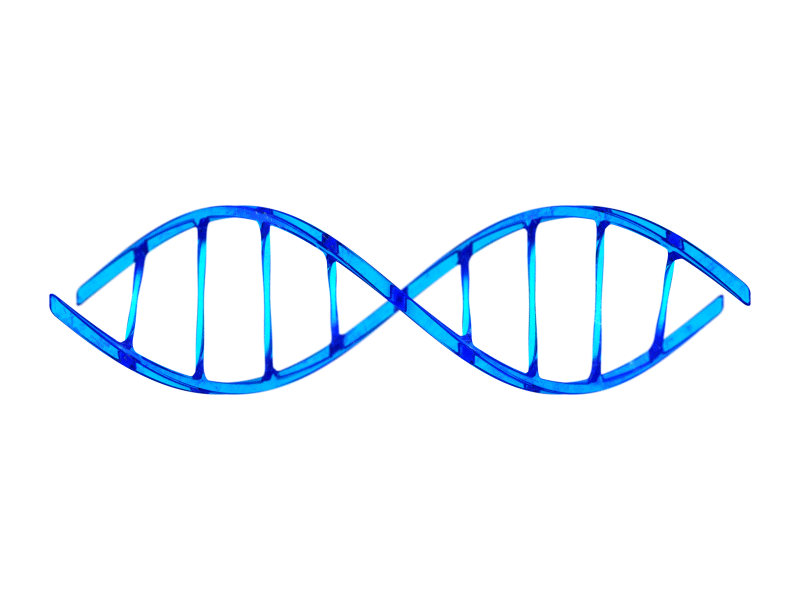Phi and its members have a pisano period if the resulting fractional numbers are truncated. If one instead rounds to the nearest number, the modular values for the first two terms are flipped compared to the 24th and 25th values as seen below.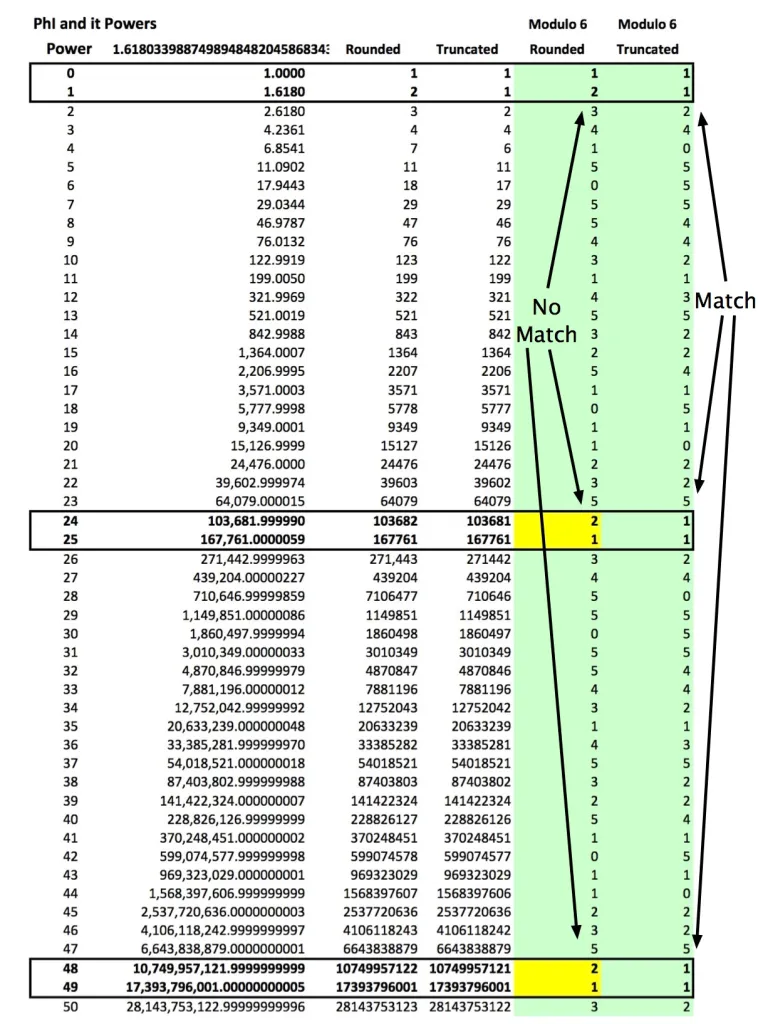Numbers fill the hexagon by spiraling until a prime number is met, then it jumps to the next minor hexagon and begins to spiral again. As the numbers continue to fill the field, the other minor hexagons from, purple, cyan and yellow.

(2112/82) − (576/82) = 24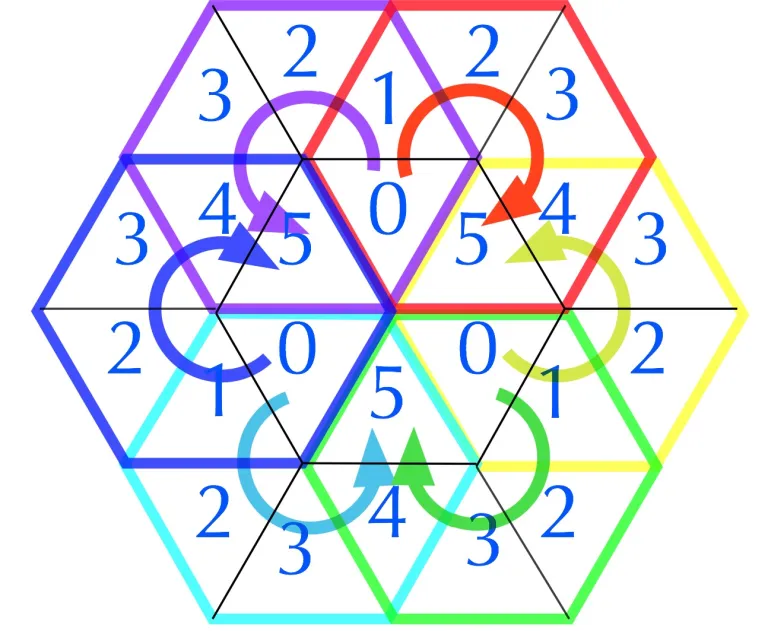``````layer | node | sub |     i    |   f
------+------+-----+----------+------                                             ---
|      |     |    1,2:1 |  71 (2,3) -------------                            |
|      |  1  +----------+                        |                           |
|  1   |     |        2 |                        |                          (5)
|      |-----+----------+                        |                           |
|      |     |        3 |                        |                           |
1   +------+  2  +----------+----                    |                          ---
|      |     |        4 |                        |                           |
|      +-----+----------+                        |                           |
|  2   |     |        5 |                        |                          (7)
289   |      |  3  +----------+                        |                           |
|     |      |     |        6 |                        | {6®}                      |
------+------+-----+----------+------      } (36)      | ↓                        ---
|      |     |     11:7√| 50/10+9 (20) ----› ¤ √ | 29=MEC30 - Δ1             |
|      |  4  +----------+                        | ↓                         |
|  3   |     |     12:8√| 9+60+40 (26) √ --      | from 329-40 √           (11)
|      +-----+----------+                  |     | ↓ lagging                 |
|      |     |     13:9√| 9+60 (27) √      | {2®} from 168 (Triangular) √    |
2   +------|  5  +----------+-----             |     | ↓ leading                ---
|      |     |    14:10√| 9+60+40 (28) √ --      | goes to 329 √             |
|      |-----+----------+                        |                           |
|  4   |     | 15,18:11√| 71 (29,30,31,32) ------                          (13)
329   |      |  6  +----------+                                                    |
|     |      |     |    19:12√| {70} (36)                                          |
------+------+-----+----------+------------------                                 ---
|      |     |    20:13√| (20-11)x10 (38) ‹- ¤ √                             |
|      |  7  +----------+                                                    |
|  5   |     |       14 |                                                  (17)
|      |-----+----------+                                                    |
|      |     |       15 |                                                    |
3   +------+  8  +----------+-----                                              ---
|      |     |       16 |                                                    |
|      |-----+----------+                                                    |
|  6   |     |    28:17√| 100(50)                                          (19)
168   |      |  9  +----------+                                                    |
|     |      |     |    29:18√| 50(68)                                             |
------|------|-----+----------+------                                             ---
``````

## Prime factor abundances

The genetic material of a cell or an organism refers to those materials found in the nucleus, mitochondria and cytoplasm, which play a fundamental role in determining the structure of cell substances and capable of self-propagating and variation.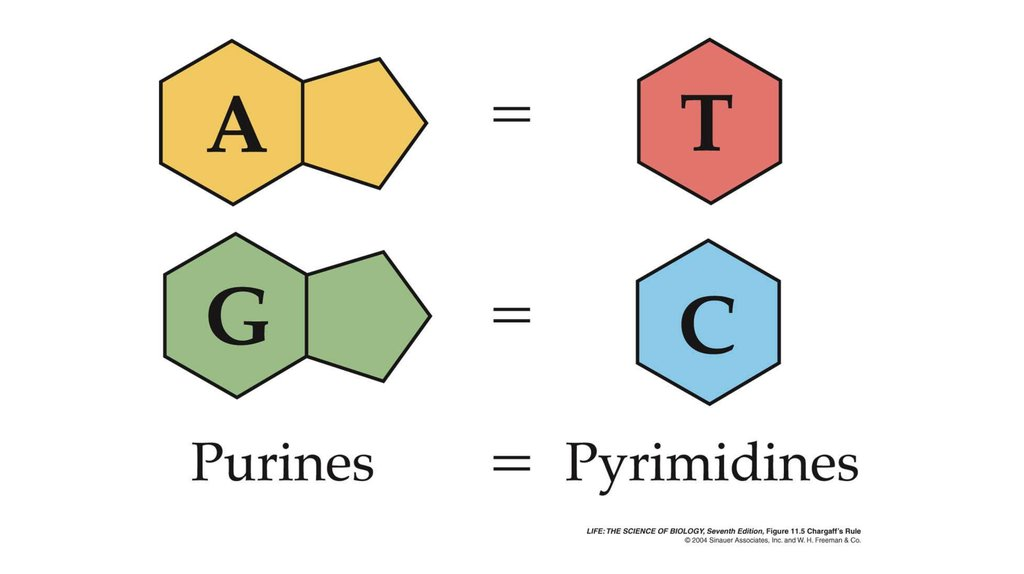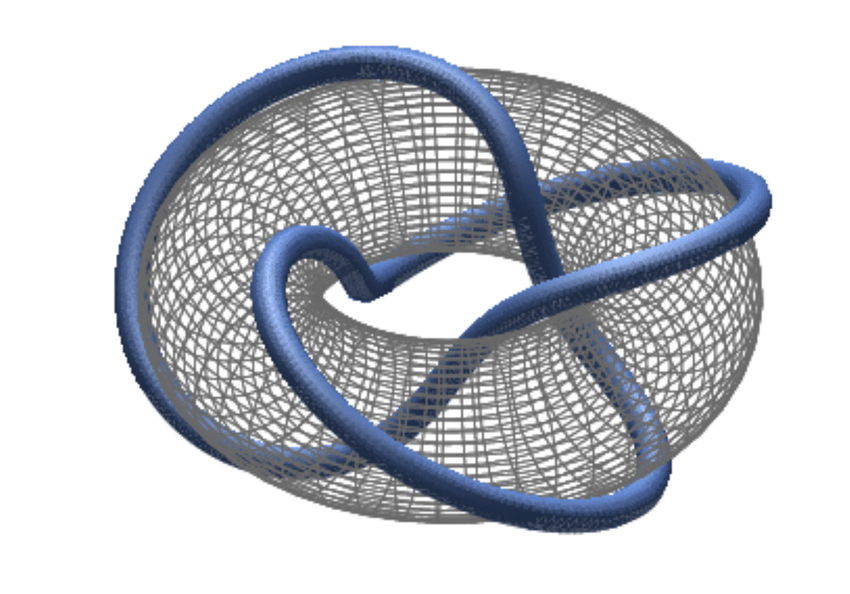Spirals following other tilings of the plane also generate lines rich in prime numbers, for example hexagonal spirals. Hexagonal number spiral with prime numbers in green and more highly composite numbers in darker shades of blue.### Ricci Flow

Finite collections of objects are considered 0-dimensional. Objects that are "dragged" versions of zero-dimensional objects are then called one-dimensional. Similarly, objects which are dragged one-dimensional objects are two-dimensional, and so on.

The basic ideas leading up to this result (including the dimension invariance theorem, domain invariance theorem, and Lebesgue covering dimension) were developed by Poincaré, Brouwer, Lebesgue, Urysohn, and Menger (MathWorld).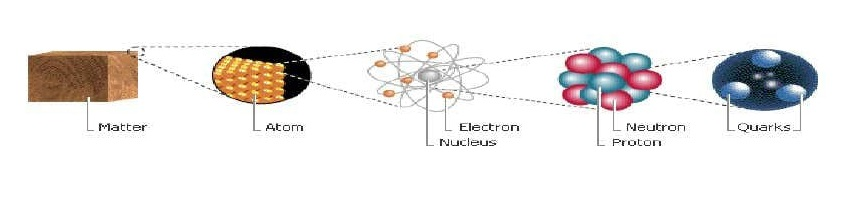The Ricci flow is a pde for evolving the metric tensor in a Riemannian manifold to make it "rounder", in the hope that one may draw topological conclusions from the existence of such "round" metrics.

Poincaré hypothesized that if such a space has the additional property that each loop in the space can be continuously tightened to a point, then it is necessarily a three-dimensional sphere (Wikipedia)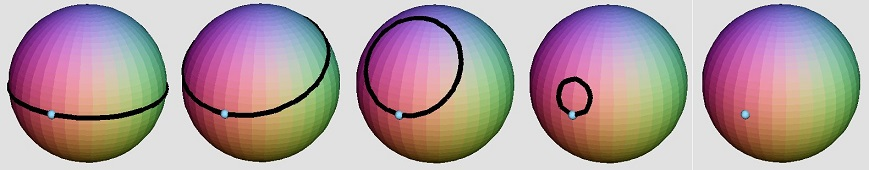The Ricci Flow method has now been developed not only in to geometric but also to the conversion of facial shapes in three (3) dimensions to computer data. A big leap in the field of AI (Artificial intelligence). No wonder now all the science leads to it.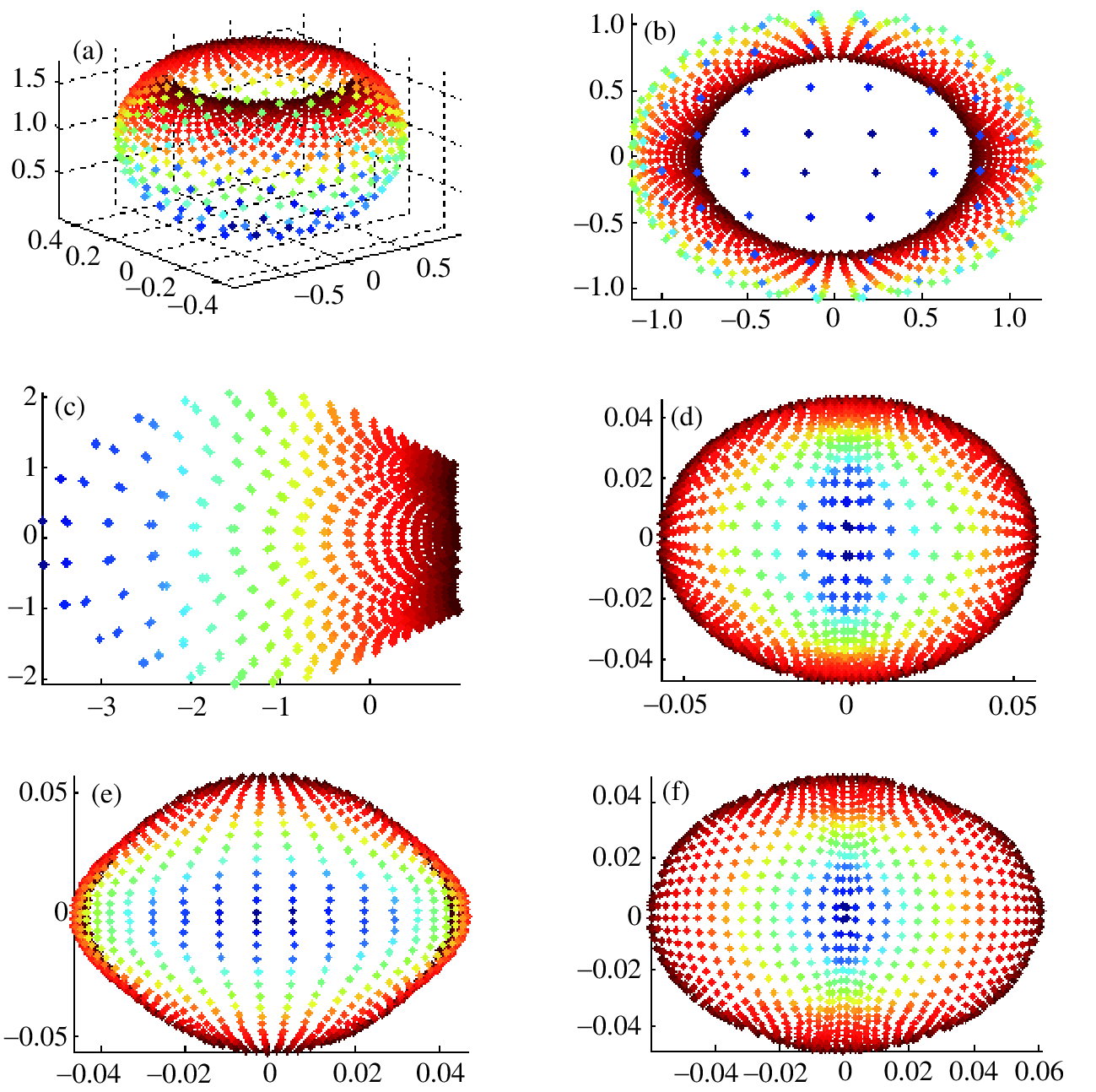So what we've discussed on this wiki is entirely nothing but an embodiment of this solved Poincare Conjecture. This is the one placed with id: 10 (ten) which stands as the basic algorithm of π(10)=(2,3,5,7).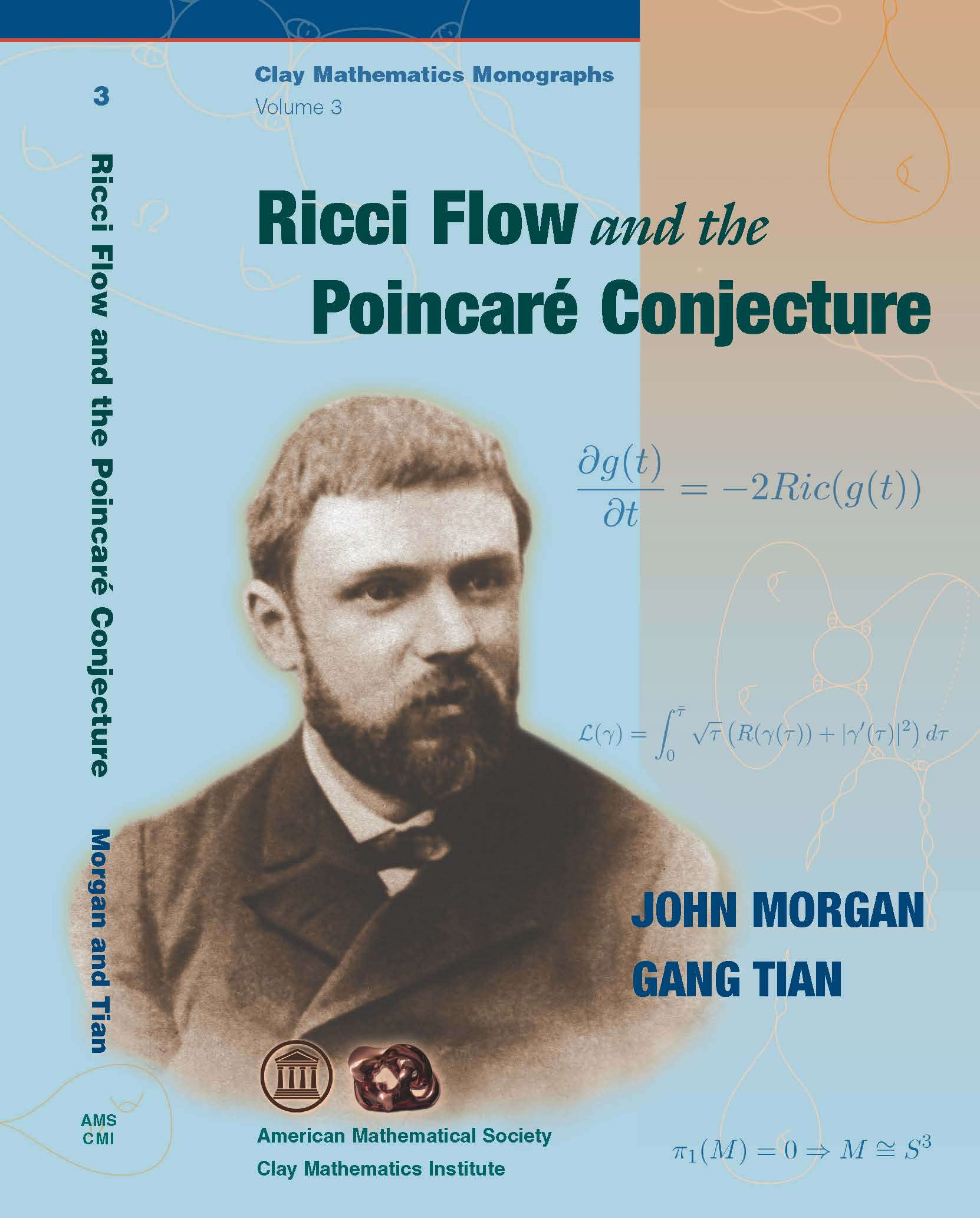Many relevant topics, such as trustworthiness, explainability, and ethics are characterized by implicit anthropocentric and anthropomorphistic conceptions and, for instance, the pursuit of human-like intelligence.

AI is one of the most debated subjects of today and there seems little common understanding concerning the differences and similarities of human intelligence and artificial intelligence (Human vs AI).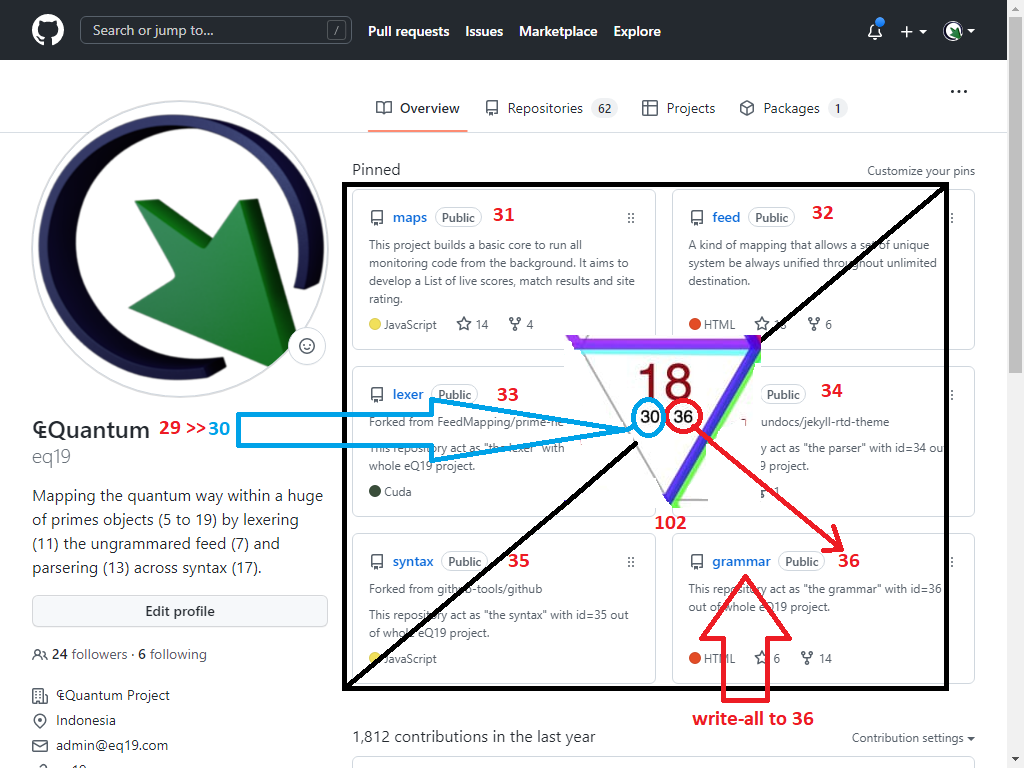Despite of any debated subjects regarding Human vs AI, the said AI would not even close to the ability of human brain without undertanding of GAP functionality between left and right of the human brain. Let's find it in details on further discussion.